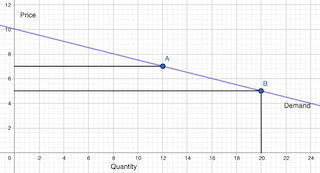# Between points A and B, what is the slope?

## Question:

Between points A and B, what is the slope?## Demand and Elasticity:

While price elasticity of demand changes depending on where on the demand curve you are, we typically saw that a flatter curve has more elastic demand. This is because quantity demanded in such cases is more sensitive to changes in price.

Become a Study.com member to unlock this answer!Price Elasticity of Demand in Microeconomics

from

Chapter 2 / Lesson 11
21K

In microeconomics, the principle of price elasticity of demand is important to understand. Learn the definition of price elasticity of demand, understand the formula and its categories, and see some calculation examples.